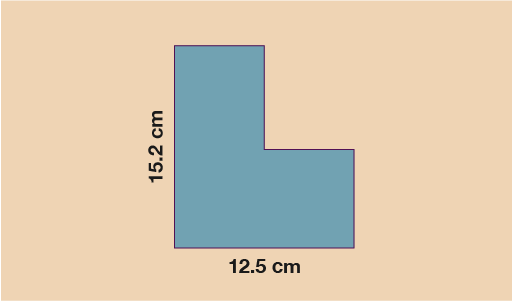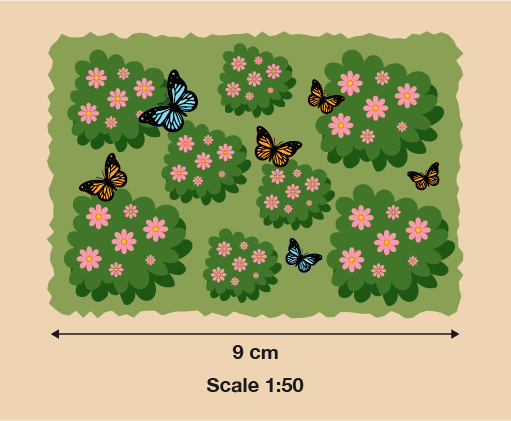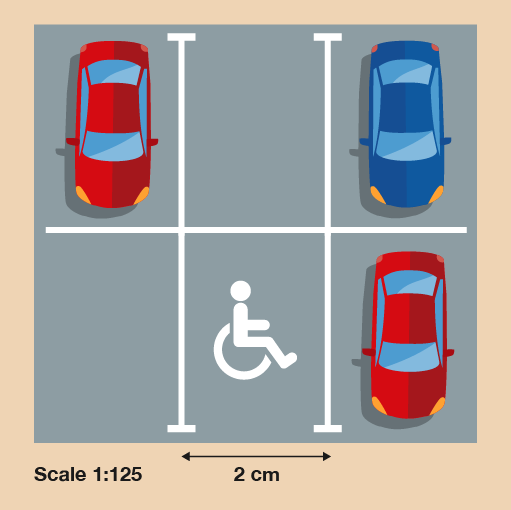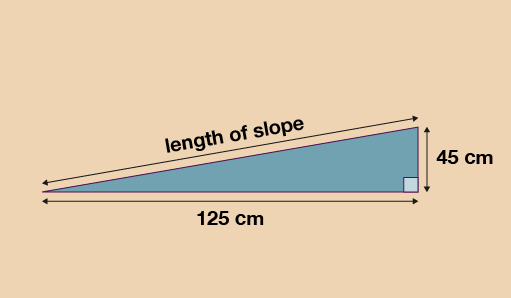Everyday maths 2 (Wales)

Start this free course now. Just create an account and sign in. Enrol and complete the course for a free statement of participation or digital badge if available.

Free course

# 5.1 Scale drawing method and problems

First watch the scale drawings example video.Skip transcript

#### Transcript

You have already developed skills in using measures and ratio. Scale drawings are just another application of this knowledge. So the good news is you already know how to do it. With this topic, it's best to dive straight in with an example.

Ahmed is going to make some home improvements. He wants to build a conservatory. Here is Ahmed's sketch of the conservatory. He uses a scale of 1 to 50. You need to draw an accurate scale drawing of the side view, using Ahmed's scale.

The scale, or ratio, we are working with is 1 to 50. This means that every 1 centimetre on the scale drawing represents 50 centimetres in real life. To be able to work out the measurements required on the scale drawing, you must first convert all measurements to centimetres. The base is 2.5 metres, which equals 250 centimetres. The shortest vertical side is 3 metres, which equals 300 centimetres. The longest vertical side is 3.75 metres, which equals 375 centimetres.

Now to work out the length of each side on the scale drawing. Since 1 centimetre on the drawing is 50 centimetres in real life, you need to divide the real-life measurement by 50 in order to work out the length required on the drawing. The base, 250, divided by 50, equals 5 centimetres. The shortest vertical side, 300, divided by 50, equals 6 centimetres. The longest vertical side, 375, divided by 50, equals 7.5 centimetres. To get the diagonal length, you simply join the tops of the two other sides together. Now work through the next few examples.

End transcript

Interactive feature not available in single page view (see it in standard view).

## Summary of method

Remember if you are given the scale drawing measurement and asked to work out the real-life size, you need to multiply.

If you are given the real-life size and asked to work out the drawing measurement, you need to divide.

## Example 1: Plan of a room

Rhodri has drawn a scale diagram of his living room. The scale he has used is 1:20.

On his diagram his living room is 30 cm long and 20 cm wide. What are the actual dimensions of his living room?

You need to work out the length and width separately.

Length

The scale he has used is 1:20, which means that everything is 20 times bigger in real life than on his diagram. You know the drawing measurement (30 cm) so you need to multiply by 20 to find the actual length of the room:

• 30 cm × 20 = 600 cm

It is a good idea to express the actual measurement in metres:

• 600 cm = 6 m

Width

You need to use the same scale of 1:20. If the width of the living room on the drawing is 20 cm then it will be 20 times as great in real life:

• 20 cm × 20 = 400 cm (400 cm = 4 m)

So Rhodri’s actual living room measures 6 m by 4 m.

Now have a go at solving these problems involving scale. Do the calculations without a calculator. You may double-check on a calculator if you need to and make sure you check your answers against ours.

## Activity 10: Scale problems

1. A scale drawing has been drawn below of a shed that a garden planner wants to build. The scale used for the drawing is 1:25.

The area that the shed will be built on is a rectangle which measures 5.1 m by 4 m. Will the shed fit into the space allocated?Figure 51 Calculating scale 1

1. You know the scale is 1:25, so 1 cm on the diagram represents 25 cm in real life. You have been given the measurements on the diagram and want to work out the actual measurements so you need to multiply.

Horizontal length:

• 12.5 × 25 = 312.5 cm = 3.125 m

Vertical length:

• 15.2 × 25 = 380 cm = 3.8 m

Since both lengths for the shed are shorter than the lengths given for the area of land, you know the shed will fit.

1. A landscaper wants to put a wild area in your garden. She makes a scale plan of the wild area:Figure 52 Calculating scale 2

What is the length of the longest side of the actual wild area in metres?

1. The length on the drawing is 9 cm, and the scale is 1:50. This means that 1 cm on the drawing is equal to 50 cm in real life. So to find out what 9 cm is in real life, you need to multiply it by 50:

• 9 × 50 = 450 cm

The question asks for the length in metres, so you need to convert centimetres into metres:

• 450 ÷ 100 = 4.5 m

The actual length of the wild area will be 4.5 m.

1. Here is a scale drawing showing one disabled parking space in a supermarket car park. The supermarket plans to add two more disabled parking spaces either side of the existing one.Figure 53 Calculating scale 3

What will be the total actual width of the three disabled parking spaces in metres?

1. You need to find out the width of three disabled parking spaces. The width of one parking space on the scale drawing is 2 cm, so first you need to multiply this by 3:

• 2 × 3 = 6 cm

The scale is 1:125. This means that 1 cm on the drawing is equal to 125 cm in real life. So to find out what 6 cm is in real life, you need to multiply it by 125:

• 6 × 125 = 750 cm

The question asks for the length in metres, so you need to convert centimetres into metres:

• 750 ÷ 100 = 7.5 m

The actual width of all three parking bays will be 7.5 m.

1. For this question you will need a pen or pencil, paper and a ruler.

Jane has a raised vegetable patch. She plans to build a slope leading up to the vegetable patch. Jane will cover the slope with grass turf.

She draws this sketch of the cross-section of the slope. The measurements indicated are the actual measurements.Figure 54 Finding the length of a slope

Jane will use a scale diagram to work out the length of the slope. She wants to use a scale of 1:10.

• a.What will the measurements of the base and height of the slope be on her diagram?

• b.Draw her scale diagram using the measurements you calculated in part (a).

(i) What will the length of the slope be on the diagram?

(ii) What will it be in real life?

1.

• a.The scale is 1:10 and you want to work out the measurements for the scale diagram, so you need to divide the real-life measurements by 10:

Base of patch = 125 ÷ 10 = 12.5 cm

Height of patch = 45 ÷ 10 = 4.5 cm

• b.  (i) Draw the scale diagram with the base measuring 12.5 cm and the height measuring 4.5 cm.

Now draw in your slope linking the end points of the base and height together. If you measure the length of the slope with a ruler it should measure around 13.2 cm on the diagram.

(ii) To work out the length of the actual slope, you need to use the scale of 1:10 again, but this time you need to multiply by 10 to work out the actual slope length:

13.2 cm × 10 = 132 cm in real life.

Your answer may vary slightly from ours but should be within a reasonable range.

## Summary

In this section you have:

• applied your ratio skills to the concept of scale plans and drawings
• interpreted scale plans.

Well done! You have now completed this section of your course. Y​ou are now ready to test the​ knowledge and skills you’ve learned in the end of session quiz.​ Good luck!​

FSM_2_CYMRU#### Find out more

*Eligibility rules apply for financial support.